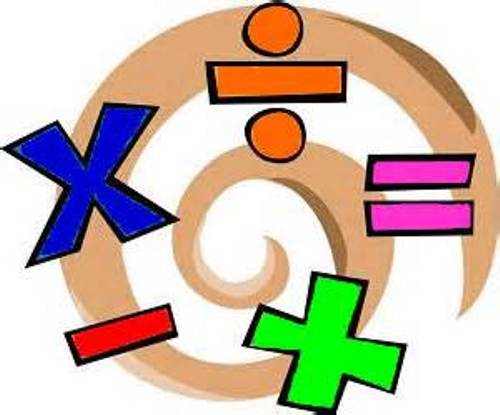# Algebra II (Pratt)

Teacher:Kerrie Pratt
Extra Help:Thursday 3-4 PM, Rm B45 or by appointment

Course Summary

Connecting math to the real world is a meaningful way for students to apply their logic and accuracy skills. Using various mathematical equations to model real life situations students will explore relationships and use them to problem solve and predict future conditions. Students will also study topics such as data analysis, probability, and series and sequences to evaluate and critique the world around them. This course will introduce more sophisticated topics originally addressed in Algebra I and Geometry courses. Topics covered include: linear models, exponential and quadratic models, radical functions and logarithmic functions.

Essential Questions:

• How do we use mathematics in our daily lives?

• What real world problems can be modeled using mathematics and how is this useful?

• In what ways do we think mathematically when problem solving?

• How do we determine the best approaches to solving a problem?

Schedule of Major Topics

Semester 1

November            Polynomial Functions
December            Polynomial Functions

Semester 2

January                Rational Exponents and Radical Functions
February              Rational Exponents and Radical Functions
March                  Exponential Functions
April                    Logarithmic Functions
May                     Linear Functions
June                     Probability (if time permits)

*Students can expect to complete a project for each unit we study.